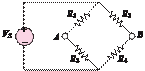### Create an Account

Home / Questions / Determine the voltage between nodes A and B in the circuit shown in Figure

# Determine the voltage between nodes A and B in the circuit shown in Figure

Determine the voltage between nodes and in the circuit shown in Figure P2.67.

V= 12 V

R1 = 11 kQ            R3 = 6.8 kQ

R2 = 220 kQ          R4 = 0.22 MQFigure P2.67

Jun 19 2020 View more View LessSubscribe To Get Solution